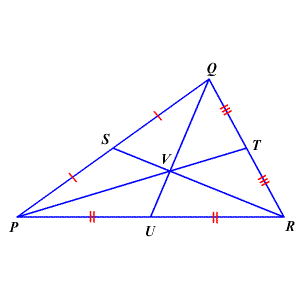# Centroid of a Triangle

The centroid of a triangle is the point where the three medians coincide.The centroid theorem states that the centroid is $\frac{2}{3}$ of the distance from each vertex to the midpoint of the opposite side.

That is,

$QV=\frac{2}{3}QU,PV=\frac{2}{3}PT,RV=\frac{2}{3}RS$